Courses

Mean Value Theorem Notes | Study Engineering Mathematics - GATE

GATE: Mean Value Theorem Notes | Study Engineering Mathematics - GATE

The document Mean Value Theorem Notes | Study Engineering Mathematics - GATE is a part of the GATE Course Engineering Mathematics.
All you need of GATE at this link: GATE

Cauchy’s Mean Value Theorem

Suppose f(x) and g(x) are 2 functions satisfying three conditions:

1. f(x), g(x) are continuous in the closed interval a <= x <= b
2. f(x), g(x) are differentiable in the open interval a < x < b and
3. g'(x) != 0 for all x belongs to the open interval a < x < b

Then according to Cauchy’s Mean Value Theorem there exists a point c in the open interval a < c < b such that:
[f(b) - f(a)] / [g(b) - g(a)] = f'(c) / g'(c)
The conditions (1) and (2) are exactly same as the first two conditions of Lagranges Mean Value Theorem for the functions individually. Lagranges mean value theorem is defined for one function but this is defined for two functions

Lagrange’s Mean Value Theorem

Suppose
f : [a, b] → R
be a function satisfying these conditions:

1. f(x) is continuous in the closed interval a ≤ x ≤ b
2. f(x) is differentiable in the open interval a < x < b

Then according to Lagrange’s Theorem, there exists at least one point ‘c’ in the open interval (a, b) such that: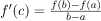We can visualize Lagrange’s Theorem by the following figure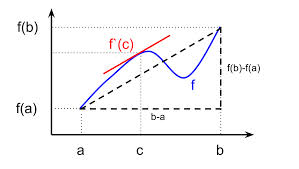In simple words, Lagrange’s theorem says that if there is a path between two points A(a, f(a)) and B(b, f(a)) in a 2-D plain then there will be at least one point ‘c’ on the path such that the slope of the tangent at point ‘c’, i.e., (f ‘ (c)) is equal to the average slope of the path, i.e.,Example: Verify mean value theorm for f(x) = x2 in interval [2, 4].
Solution: First check if the function is continuous in the given closed interval, the answer is Yes. Then check for differentiability in the open interval (2, 4), Yes it is differentiable.
f'(x) = 2x
f(2) = 4
and f(4) = 16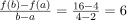Mean value theorem states that there is a point c ∈ (2, 4) such that
f' (c) = 6
But
f' (x) = 2x
which implies c = 3. Thus at c = 3 ∈ (2, 4), we have
f' (c) = 6

Rolle’s Mean Value Theorem

Suppose f(x) be a function satisfying three conditions:

1. f(x) is continuous in the closed interval a ≤ x ≤ b
2. f(x) is differentiable in the open interval a < x < b
3. f(a) = f(b)

Then according to Rolle’s Theorem, there exists at least one point ‘c’ in the open interval (a, b) such that:
f ‘(c) = 0
We can visualize Rolle’s theorem from the figure(1)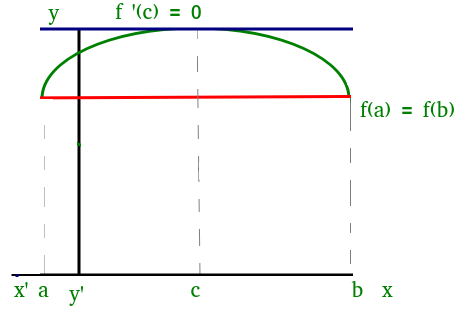Figure(1)

In the above figure the function satisfies all three conditions given above. So, we can apply Rolle’s theorem, according to which there exists at least one point ‘c’ such that:

f ‘ (c) = 0
which means that there exists a point at which the slope of the tangent at that is equal to 0. We can easily see that at point ‘c’ slope is 0.
Similarly, there could be more than one points at which slope of tangent at those points will be 0. Figure(2) is one of the example where exists more than one point satisfying Rolle’s theorem.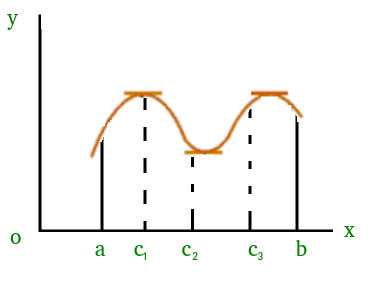Figure(2)

The document Mean Value Theorem Notes | Study Engineering Mathematics - GATE is a part of the GATE Course Engineering Mathematics.
All you need of GATE at this link: GATEUse Code STAYHOME200 and get INR 200 additional OFF Use Coupon CodeEngineering Mathematics

54 docs|21 tests

Track your progress, build streaks, highlight & save important lessons and more!

,

,

,

,

,

,

,

,

,

,

,

,

,

,

,

,

,

,

,

,

,

;